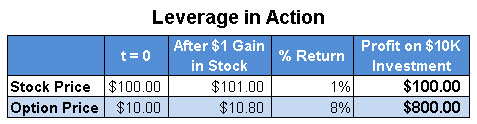# How the Leverage Ratio Predicted a Yandex NV (YNDX) Winner

## Our 'leverage ratio' lets you calculate how much bang you can expect for your option-trading buck

Editor-in-Chief
Aug 3, 2015 at 10:23 AM

The following is a reprint of the market commentary from the August 2015 edition of The Option Advisor, published on July 23. For more information or to subscribe to The Option Advisor, click here.

Traders tend to learn about the concept of leverage fairly early on in the course of their options education, as this is one of the key benefits of trading puts and calls instead of stocks. In the simplest terms, "leverage" simply refers to the fact that options players have the ability to generate profits many times greater than their initial investment -- and many times greater than those available to shareholders -- due to the reduced cost of entry on options contracts, relative to an equivalent number of shares in the underlying stock.

While it's easy to discuss this mathematical benefit on a theoretical basis, it would be even better if options players were able to define, in concrete terms, what specific degree of leverage they might expect to obtain from a put or call purchase prior to entering the trade order with their broker. To that end, we've recently been relying on a calculation we call the "leverage ratio" to inform our options trading decisions.

The leverage ratio is calculated as follows:

(stock price * option delta)/option price

The resulting number is the multiple by which your return on the option trade can be expected to exceed a comparable stock trade. (Delta, for those unfamiliar with the concept, is a metric reflecting how much value an option will lose or gain for each one-point move in the underlying stock.)

Say we have Stock XYZ trading at \$100, and we're considering buying an in-the-money call option on that stock. The option in question has a delta of 80%, and is asked at \$10. Multiplying the stock price of \$100 by the delta of 0.8, then dividing that product by the option price of \$10, results in a leverage ratio of 8.00. This tells us the investment in this option will yield 8 times the profit as a comparable investment in the stock.

The table below breaks down this outcome in more detail. We see in the "time equals zero" column that the stock is at \$100 per share, and the option is priced at \$10. After the stock gains one point of value, it's worth \$101 per share. Based on the delta of 80%, our \$10 option will be worth \$10.80 following this one-point move in the stock.

Therefore, while the stock gained 1% (from \$100 to \$101), our option gained 8% (from \$10 to \$10.80). As the final column in the table shows, if we had invested \$10,000 in the stock, we would have had a profit of \$100 -- but if we invested that same \$10,000 in the options, we would have a profit of \$800.To further bring this calculation to life, here's an example of a recent trade from our Weekend Trader Series option recommendation service. On June 15, we told subscribers to buy to open the Yandex NV (NASDAQ:YNDX) August 19 put. We calculate entry prices for this particular service by using the average of the high and low prices during the day -- which was \$2.33 for the option, and would have been \$17.35 on the stock. Meanwhile, the option's delta was negative 67.8%. Based on these figures, our leverage ratio arrived at 5.05 -- or (17.35 * 0.678)/2.33.

Our Weekend Trader Series recommendations target 100% gains. Since the leverage ratio of 5.05 tells us the options will gain 5.05 times the stock's gain, we can divide our target profit of 100 by 5.05 to determine what kind of move we need in the underlying shares in order to achieve this return. In this case, 100/5.05 = 19.80. In other words, we'd need a 19.8% drop in YNDX shares for our put to achieve its target profit of 100%.

(In this manner, the leverage ratio is useful not only for replacing the often-nebulous concept of "leverage" with a concrete number, but also for allowing traders to determine if the stock move necessary to yield desired results on the option is feasible within the framework of the equity's current technical situation.)

YNDX shares went on to hit a low of \$13.90 on July 7, representing a decline of 19.88% from the average stock entry price of \$17.35. Multiplying that 19.88% move by our leverage ratio of 5.05 yields a theoretical return of 100.4% on the option -- pretty close to our actual gain of 101.7% at closeout.

Trader: Sell These 8 Stocks in 2021